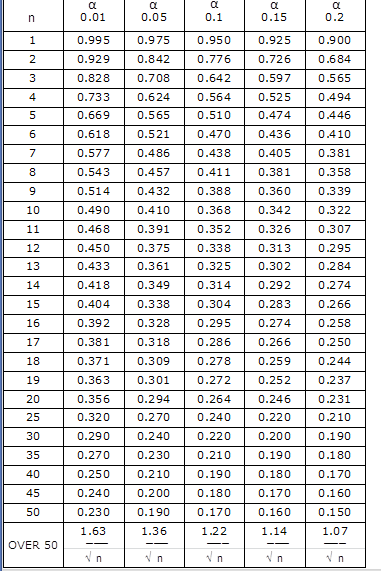# Why does the Kolmogorov distribution table have entries for $n$?

In my textbook it is said, that if the hypothesis of same population distributions is true, then $$\sqrt{n}D\rightarrow K$$ in distribution, where $$D$$ is the Kolmogorov-Smirnov statistic, and $$K$$ is Kolmogorov distribution. So, we are interested in the value of $$\sqrt{n}D$$. So why does the tables for Kolmogorov distribution have also entries for $$n$$, and then only for $$n$$ larger than $$50$$, it is given in value$$/\sqrt{n}$$ units? To me, it seems that we only need one row, instead of one row for each $$n$$. For example, this table:• Plug the smaller values of $n$ into the formulas, like $1.63/\sqrt{n},$ and compare what you get with what's in the table. – whuber May 28 at 19:47

The arrow in the expression $$\sqrt{n}D\rightarrow K$$ means that $$K$$ is the asymptotic distribution of $$\sqrt{n}D$$, and you can consider it to be a good approximation of your test statistic's distribution only when $$n$$ is big enough. Hence the need for a table that considers the distribution of $$D$$ when $$n$$ is little.